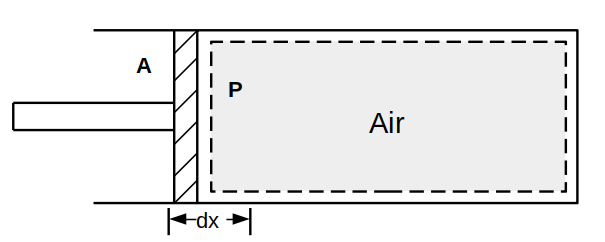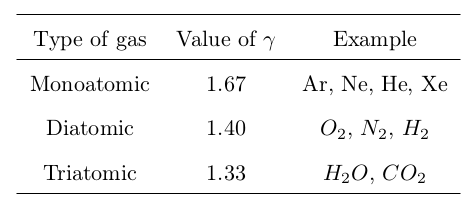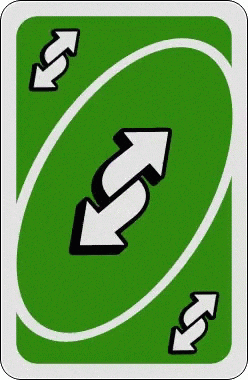Search
•rameez badhurshah

# Thermodynamics - Key Ingredients

Updated: Jul 21, 2021

What are the basic concepts and definitions of thermodynamics? What are the different processes in which a thermodynamic change can be brought about?

Before we start, let us understand what thermodynamics means? The literal meaning of thermodynamics translates to “heat motion”, implying the study of heat in motion. Alternatively, the fascinating science of exchange between heat energy and mechanical energy can be understood as thermodynamics. For most people, thermodynamics has always been a subject with tons of equations to remember. This has in the past, scared young thermodynamics and kept them off the bay from the subject. Here, we have tried to stick to a text-based understanding with minimal use of equations. Let’s quickly summarize a few terminologies which will keep occurring throughout the course of this subject.

## Definitions in thermodynamics

1. System: Any matter or space of interest. The system can be further categorized as:2. Surrounding: Matter that lies away from the system.

3. Boundary: A real/ imaginary surface separating the system and surroundings.

4. Universe: The system and surrounding together constitute the universe5. Intensive Quantity: Any quantity independent of mass or the extent of the system. Examples: Density, Atmospheric pressure.

6. Extensive Quantity: Quantity dependent on mass. Example: Total heat

7. Total Quantity: Quantity calculated for total mass. Example: Total heat transfer (Q).

8. Specific Quantity: Quantity calculated for unit mass or unit volume. Example: Specific heat transfer (q), Specific volume (v = V/m)

9. Point Function/ Property: Quantity dependent only on initial and final states and independent of the path chosen or process. Example: Change in potential energy (\$\Delta PE = PE_2 - PE_1\$)

.10. Path Function: Quantity dependent on the path chosen along with the initial and the final points. Example: Heat transfer

11. State: It is the condition or status of the system and is specified by a set of thermodynamic properties.

12. Process: Process is the path between the initial and the final state. It could be also termed as a combination of states to facilitate the system's transition from initial to final condition.

13. Cycle: Combination of processes so arranged such that the end of one process marks the beginning of another and the end of the last process is the beginning of the first process.14. Temperature: Degree of hotness or coldness. It's measured either in C (Degree Celsius), F (Degree Fahrenheit) or K (Kelvin).

15. Heat transfer: Energy transfer arising due to temperature gradient. Heat transfer to the system is positive, heat transfer from the system is negative. Work done on the system is negative while work done by the system is positive.

## Ideal Gas Law

The most basic gas law which relates the various thermodynamic variable such as pressure, volume and temperature is the Ideal gas law. Mathematically,where P is absolute pressure in kPa, V is the volume in m^3, m is the mass of gas in kg, R is the gas constant (kJ/kg K) and T is the temperature in K.where Ru is the universal gas constant and n is the volume in kmol.

## Scope of Thermodynamics

Thermodynamics deals with the system of thermodynamic equilibrium. The thermodynamic equilibrium of the system is the simultaneous equilibrium of mechanical equilibrium (uniform pressure), thermal equilibrium (uniform temperature) and chemical equilibrium (uniform composition).

## Closed System Analysis

### WorkA cylinder enclosing a gas attached with a piston freely movable in X-direction

Consider a cylinder with a movable piston of area (A) resembling a closed system. The cylinder is filled with a gas of Pressure (P). For the small displacement (dx) of the piston inside the cylinder, the small work done (W) by the piston on the gas will be force (F) times displacement. MathematicallyFor total work,Note: As work done depends on the path of the process, work is not a property.

### Internal energyA system taking in heat and doing work

Internal energy is the energy that the system itself uses up. In simple words, it is the difference between the heat supplied and the work done by the system.

## Types of processes

When a system undergoes a change from one equilibrium state to another equilibrium state, this change is termed a process while the series of states that the system experiences is called the path of the process. A process can be described completely by specifying the initial state, the final state, the path and the system's interaction with the surroundings. The various types of processes are:

1. Isobaric/Isopiestic process: A process with constant pressure. Thus, from the ideal gas equation, T is proportional to V (Charles 1st law).

2. Isochoric/Isometric process: A process with constant volume. Thus, from the ideal gas equation, T is proportional to P (Charles 2nd law). Work done in the isochoric process is zero.3. Isothermal process: A process with a constant temperature. Thus, P will be inversely proportional to V (Boyle's law).

4. Adiabatic process: A process with no heat transfer.Value of ratio of specific heats for different type of gas

5. Reversible process: The state of the system can be reversed back to the initial state by reversing the effects without leaving any trace.6. Irreversible process: The state of the system cannot be reversed back to the initial state by reversing the effects and without leaving any trace of the process. \par

Note: All real processes are irreversible. Major reasons for irreversibility:

1. Mechanical friction

2. Sudden expansion in vacuum

3. Heat transfer with temperature difference

4. Unwanted heat losses

7. Quasi-static process: An infinitesimally slow process. Approximated as a reversible process.

## History of thermodynamics

The fundamentals of thermodynamics have always been in existence in the universe. However. only the successful demonstration of the first steam engines in 1697 by Thomas Savery and in 1712 by Thomas Newcomen paved the path for studying thermodynamics as a science subject.

The focus of initial thermodynamic studies was to turn these slow inefficient engines into more efficient ones. The term thermodynamics was coined by Lord Kelvin in 1849 while the first textbook on this subject was not published until 1859 by Prof. William Rankine (University of Glasgow). Interestingly. the first and the second laws of thermodynamics evolved simultaneously during the same period 1850s.

## Summary

• We have introduced all the basic terminologies that keep occurring repeatedly in the subject of thermodynamics.

• We also looked at the Ideal gas equation and the various process that emerge out of this basic law.

• We also see, why it's nearly impossible to have a process that is reversible in nature.

Stay tuned for your latest articles by subscribing us!